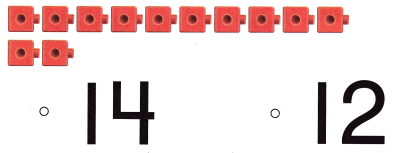# Texas Go Math Kindergarten Lesson 7.4 Answer Key Count and Write 13 and 14

Refer to our Texas Go Math Kindergarten Answer Key Pdf to score good marks in the exams. Test yourself by practicing the problems from Texas Go Math Kindergarten Lesson 7.4 Answer Key Count and Write 13 and 14.

## Texas Go Math Kindergarten Lesson 7.4 Answer Key Count and Write 13 and 14

Explore

DIRECTIONS: Count and tell how many. Trace and write the numbers and the words.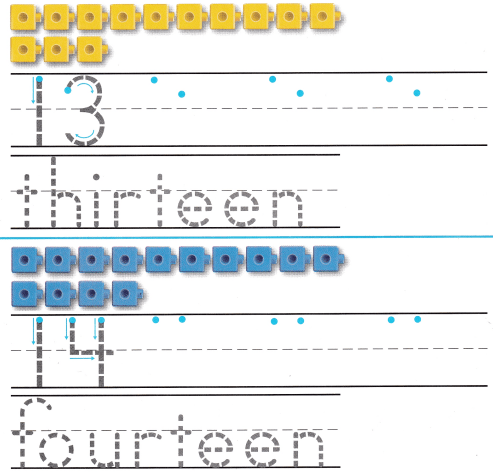Explanation:
Number of yellow cubes = 13 or Thirteen.
Number of blue cubes = 14 or Fourteen.

Share and Show

DIRECTIONS: 1. Count and tell how many. Practice writing the number. 2. Count and tell how many. Write the number. 3. Look at the squares in Exercise 2. Write how many blue squares. Write how many red squares. Write how many squares in all.

Question 1.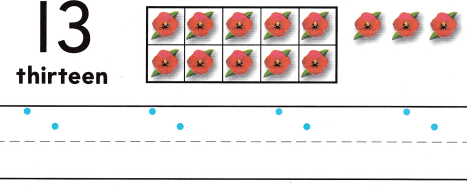Explanation:
Number of flowers = 13 or Thirteen.

Question 2.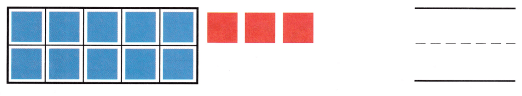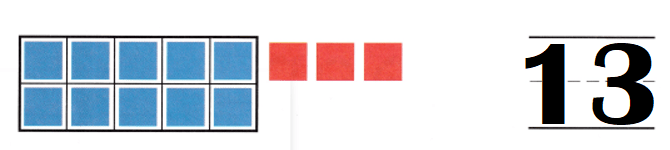Explanation:
Number of blue cubes = 10.
Number of red cubes = 3.
Total number of cubes = Number of blue cubes  + Number of red cubes
= 10 + 3
= 13.

Question 3.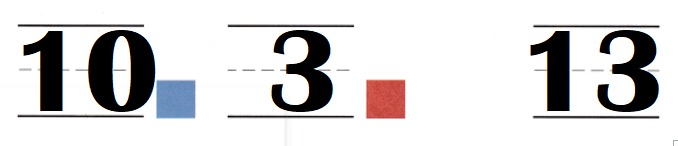Explanation:
Number of blue cubes = 10.
Number of red cubes = 3.
Total number of cubes = Number of blue cubes  + Number of red cubes
= 10 + 3
= 13.

DIRECTIONS: 4. Count and tell how many. Practice writing the number. 5. Count and tell how many. Write the number. 6. Look at the squares in Exercise 5. Write how many red squares. Write how many blue squares. Write how many squares in all.

Question 4.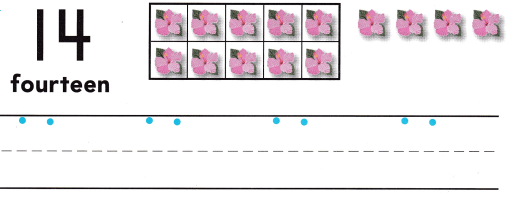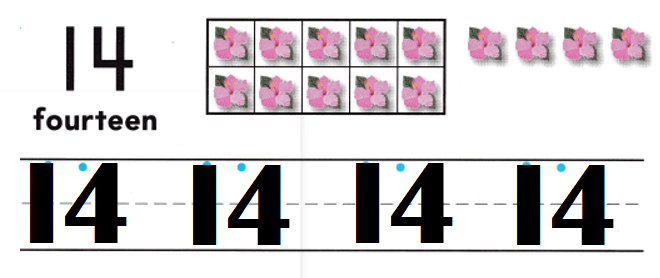Explanation:
Number of flowers = 14 or Fourteen.

Question 5.Explanation:
Number of red cubes = 10.
Number of blue cubes = 4.
Total number of cubes = Number of red cubes  + Number of blue cubes
= 10 + 4
= 14 or Fourteen.

Question 6.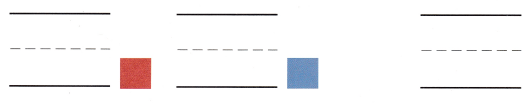Explanation:
Number of red cubes = 10.
Number of blue cubes = 4.
Total number of cubes = Number of red cubes  + Number of blue cubes
= 10 + 4
= 14 or Fourteen.

DIRECTIONS: 7. Tb picked 13 purple flowers. Draw more purple flowers to show how many she picked. 8. Calvin picked 14 red flowers. Draw more red flowers to show how many he picked. 9. Count the cubes. Write the number.

Problem Solving
Question 7.Explanation:
Number of purple flowers given = 10.
Total number of purple flowers = 13 or Thirteen.
Number of purple flowers missing = Total number of purple flowers – Number of purple flowers given
= 13 – 10
= 3.

Question 8.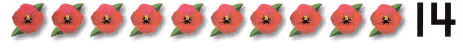Explanation:
Number of red flowers given = 10.
Total number of red flowers = 14 or Fourteen.
Number of red flowers missing = Total number of red flowers – Number of red flowers given
= 14 – 10
= 4.

Question 9.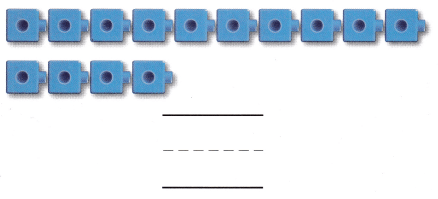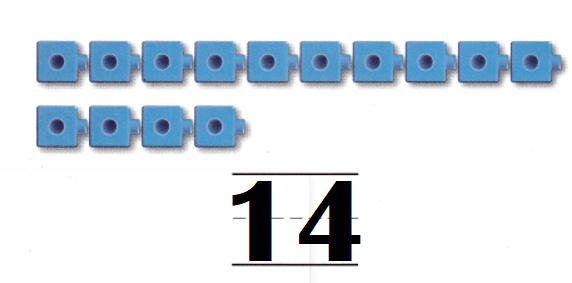Explanation:
Number of blue cubes = 14 or Fourteen.

### Texas Go Math Kindergarten Lesson 7.4 Homework and Practice Answer Key

DIRECTIONS: 1-2. Count and tell how many. Practice writing the number.

Question 1.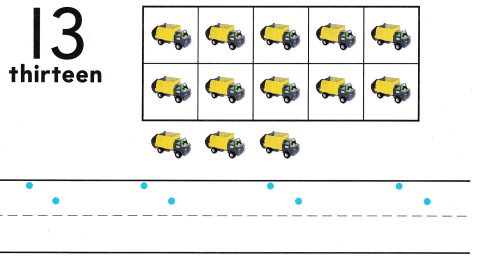Explanation:
Number of lorries = 13 or Thirteen.

Question 2.Explanation:
Number of red cookies = 14 or Fourteen.

DIRECTIONS: Choose the correct answer 3-5. Count the cubes. How do you write that number?

Lesson Check
Question 3.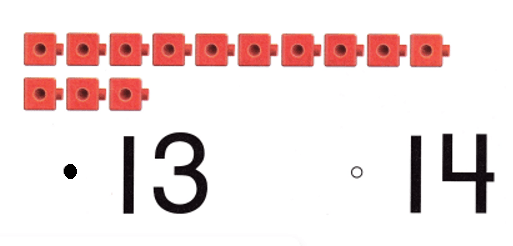Explanation:
Number of red cubes = 13 or Thirteen.

Question 4.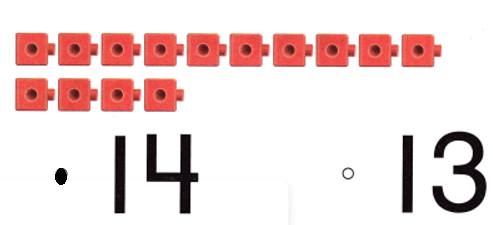Explanation:
Number of red cubes = 14 or Fourteen.

Question 5.